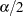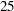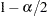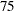OUTPUT Statement
OUTPUT <OUT=SAS-data-set> <keyword <=name> > ...<keyword <=name> > ;

The OUTPUT statement creates a new SAS data set that saves diagnostic measures calculated for the selected model. If you do not specify a keyword, then the only diagnostic included is the predicted response.

All the variables in the original data set are included in the new data set, along with variables created in the OUTPUT statement. These new variables contain the values of a variety of statistics and diagnostic measures that are calculated for each observation in the data set. If you specify a BY statement, then a variable _BY_ that indexes the BY groups is included. For each observation, the value of _BY_ is the index of the BY group to which this observation belongs. This variable is useful for matching BY groups with macro variables that PROC GLMSELECT creates. See the section Macro Variables Containing Selected Models for details.

If you have requested-fold cross validation by requesting CHOOSE=CV, SELECT=CV, or STOP=CV in the MODEL statement, then a variable _CVINDEX_ is included in the output data set. For each observation used for model training the value of _CVINDEX_ isif that observation is omitted in forming the ith subset of the training data. See the CVMETHOD= for additional details. The value of _CVINDEX_ is 0 for all observations in the input data set that are not used for model training.

If you have partitioned the input data with a PARTITION statement, then a character variable _ROLE_ is included in the output data set. For each observation the value of _ROLE_ is as follows:

 _ROLE_ Observation Role TEST testing TRAIN training VALIDATE validation

If you want to create a permanent SAS data set, you must specify a two-level name (for example, libref.data-set-name).

For more information on permanent SAS data sets, refer to the section "SAS Files" in SAS Language Reference: Concepts.

Details on the specifications in the OUTPUT statement follow.

keyword <=name>

specifies the statistics to include in the output data set and optionally names the new variables that contain the statistics. Specify a keyword for each desired statistic (see the following list of keywords), followed optionally by an equal sign, and a variable to contain the statistic.

If you specify keyword=name, the new variable that contains the requested statistic has the specified name. If you omit the optional =name after a keyword, then the new variable name is formed by using a prefix of one or more characters that identify the statistic. For residuals and predicted values, the prefix is followed by an underscore (_), followed by the dependent variable name.

The keywords allowed and the statistics they represent are as follows:

PREDICTED | PRED | P

predicted values. The prefix for the default name is p.

RESIDUAL | RESID | R

residual, calculated as ACTUALPREDICTED. The prefix for the default name is r.

When you also use the MODELAVERAGE statement, the following keywords and the statistics that they represent are also available:

LOWERpercentile of the sample predicted values. By default,, which yields theth percentile. You can change the value ofby using the ALPHA= option in the MODELAVERAGE statement. The default name is LOWER.

MEDIAN

median of the sample predicted values. The default name is median.

SAMPLEFREQ | SF

sample frequencies. For theth sample, a column that contains the frequencies used for that sample is added. The name of this column is formed by appending an indexto the name that you specify. If you do not specify a name, then the default prefix is sf.

SAMPLEPRED | SP

sample predictions. For theth sample, a column that contains the predicted values produced by the model selected for that sample is added. The name of this column is formed by appending an indexto the name that you specify. If you do not specify a name, then the default prefix is sp.

STANDARDDEVIATION | STDDEV

standard deviation of the sample predicted values. The default name is stdDev.

UPPERpercentile of the sample predicted values. By default,, which yields theth percentile. You can change the value ofby using the ALPHA= option in the MODELAVERAGE statement. The default name is UPPER.

OUT=SAS data set

specifies the name of the new data set. By default, the procedure uses the DATAn convention to name the new data set.